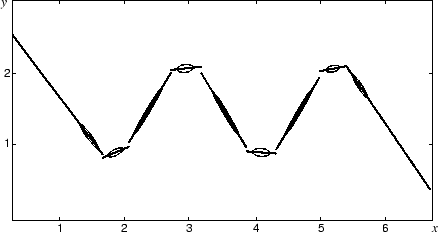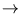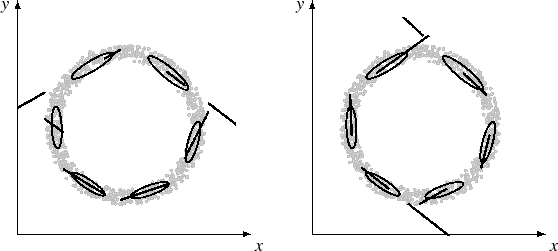Next: 4.4 Image Completion Up: 4. Abstract recurrent neural Previous: 4.2 Recall algorithm

# 4.3 Function approximation on synthetic data

To demonstrate the working of the recall algorithm, it was tested on two synthetic pattern distributions, a noisy sine wave and a noisy circle. For both distributions, the mixture of local PCA was gained by using the algorithm MPPCA-ext (section 3.3). However, the alternative algorithm NGPCA (section 3.2) could have been also used; the results were similar.

The sine-wave distribution is composed of 800 points. The mixture model contained nine ellipses with two principal components each. Figure 4.3 shows the result of the recall if the x-coordinate was given. The recall is locally linear and discontinuities occur between the changes from one ellipse to the next. On a global scale, the sine-wave is correctly restored.The second test illustrates the two advantages over feed-forward networks, like multi-layer perceptrons. The distribution consists of 1000 points arranged in a noisy circle with radius 1.0 (figure 4.4). It was approximated by six ellipses with two eigenvectors each. Figure 4.4 shows the results of the recall for two different directions ( xy and yx) using the same mixture of local PCA. The mapping in both directions is redundant (one-to-many). Nevertheless, the algorithm finds a valid solution that lies on the distribution of training patterns for input values in the training domain. Here, the solution jumps between the two semicircles. In contrast, a multi-layer perceptron would average over redundant solutions, and thus, it would learn to produce a line going through the middle of the circle.Next: 4.4 Image Completion Up: 4. Abstract recurrent neural Previous: 4.2 Recall algorithm
Heiko Hoffmann
2005-03-22